# How to Use the Excel COUNTA FunctionClaudia Buckley

The Excel COUNTA function counts all cells with data — whether that data is text, numbers, formulas, Booleans, or error values.

## Comparing functions in the COUNT family

To understand what gets counted and what doesn’t when using the different Excel COUNT functions, the following diagram will be useful.

If you're looking for more resources on how to count in Excel, check out our guides to the COUNT, COUNTIF and UNIQUE functions.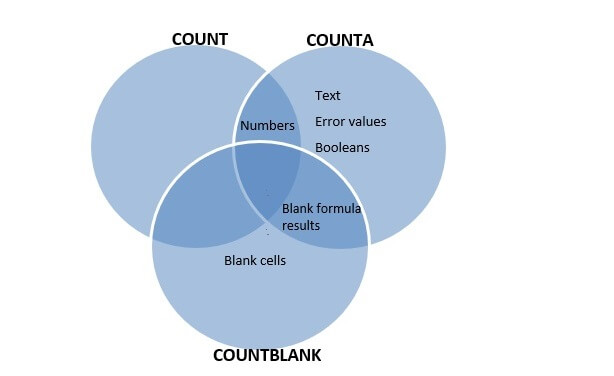Clearly, COUNTA is more inclusive than the COUNT function, which only counts cells containing numeric data. The COUNTA syntax is simply:

``=COUNTA(value1, [value2],...)``

So COUNTA will count all non-blank cells within a range and will tell us exactly how many cells have data. The only thing not counted by the COUNTA function are cells that have absolutely no information.

In the example below, COUNTA returns a value of 6 non-empty cells since it counts the text, numbers, and error values within the range B2 to B8.

``=COUNTA(B2:B8)``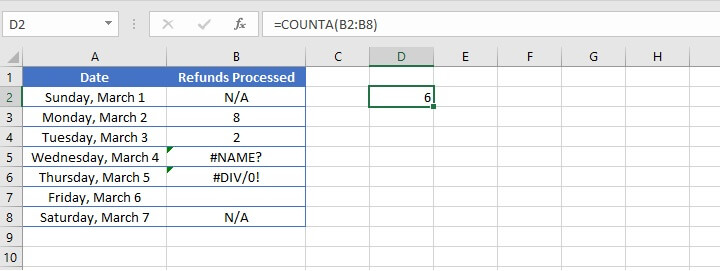Use this free Excel file to practice along with the COUNTA tutorial.

## My COUNTA function is not working

There may be times when your COUNTA formula gives an unexpected result. It is important to remember that a cell that appears to be empty may, in fact, contain a space or a formula that gives it an appearance of being empty. The COUNTA function will include these cells in its non-empty cell count also.

This is shown in the following example. The same formula, =COUNTA(B2:B8), is entered in cell D2, returning a result of 6, even though cells B5 and B6 appear to be empty.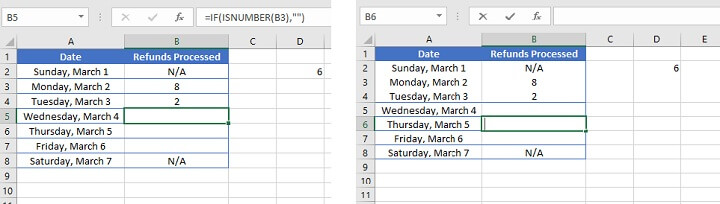The image above shows that cell B5 contains a formula that returned a blank cell, and cell B6 contains a space, that is a text value. Therefore, only cell B7 was excluded from the COUNTA tally, bringing the total to 6 cells with data. If this is an unexpected or undesired result, see below for how to resolve these issues.

## Do not count cells that are visibly empty

### Cells with formulas returning a blank result

Referring back to the Venn diagram above, it is easier to visualize that in order to count only cells that contain a visible value, we need to count all the cells within the relevant range. And then subtract the number returned by the COUNTBLANK formula in order to exclude cells containing a formula that returns a blank result.

To count all cells within a range, we can use the formula:

``=ROWS(array)*COLUMNS(array)``

Therefore, in the example below, where cell B5 contains a formula but the cell appears blank, using the following hack will count everything the COUNTA function does, minus the formulas returning a blank as the result.

``=ROWS(B2:B8)*COLUMNS(B2:B8)-COUNTBLANK(B2:B8)``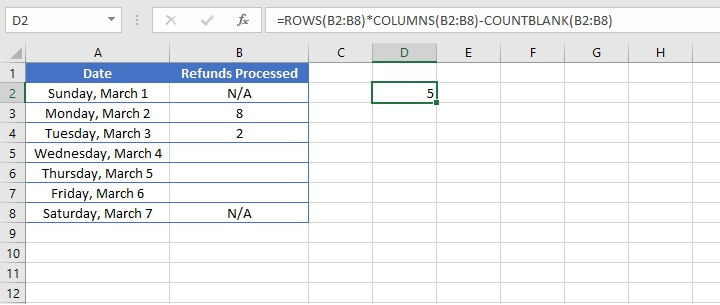The cells counted above are: cells with text, numbers, and a space (B6).

### Cells containing only a space

To remove cells that only contain a space (maybe they were entered in error), follow these steps:

1. Highlight the range in question and go to the Find and Replace dialog box (Ctrl+H or Cmd+H).
2. In the ‘Find what’ field, type a single space.
3. In the ‘Replace with’ field, ensure that nothing is typed.
4. Go to Options and check the ‘Match entire cell contents’ box.
5. Click Replace All.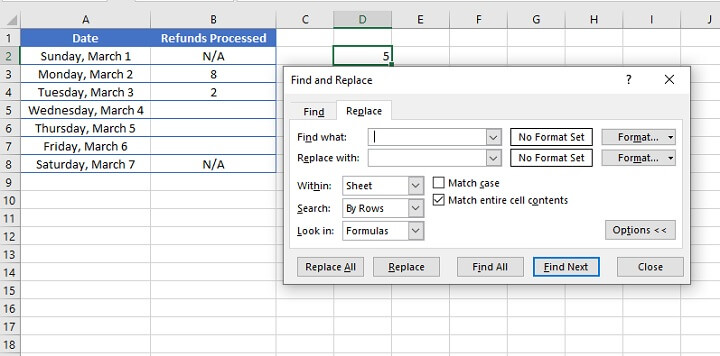Cells with only a single space will have that space removed, and a pop-up box telling you how many replacements were made will appear.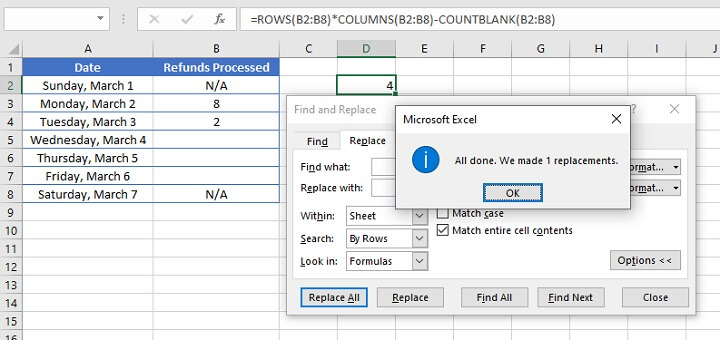Now the count in cell D2 is adjusted to 4, to reflect the removal of the space from cell B6.

These two possibilities aside, the Excel COUNTA function is usually the go-to formula to count cells containing values and/or data.

If you're looking for more resources on how to count in Excel, check out our guides to the COUNT, COUNTIF and UNIQUE functions.

To learn more essential Excel skills to boost your productivity, try the free GoSkills Excel in an Hour course today.